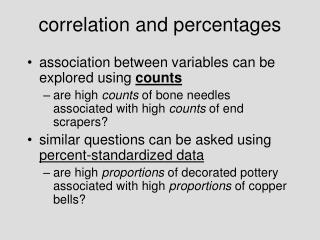DownloadDownload Presentationcorrelation and percentages

# correlation and percentages

Download Presentation## correlation and percentages

- - - - - - - - - - - - - - - - - - - - - - - - - - - E N D - - - - - - - - - - - - - - - - - - - - - - - - - - -
##### Presentation Transcript

1. correlation and percentages • association between variables can be explored using counts • are high counts of bone needles associated with high counts of end scrapers? • similar questions can be asked using percent-standardized data • are high proportions of decorated pottery associated with high proportions of copper bells?

2. but… • these are different questions with different implications for formal regression • percents will show some correlation even if underlying counts do not… • ‘spurious’ correlation (negative) • “closed-sum” effect

3. 10 vars. 5 vars. 3 vars. 2 vars. matrix(round(rnorm(100, 50, 15), nrow=10)))

4.  original counts  %s (10 vars.)  %s (5 vars.)  %s (3 vars.)  %s (2 vars.)

5. original counts %s 10 vars. %s 5 vars. %s 3 vars. %s 2 vars.

6. outliers

7. including outliers in regression analyses is usually a bad idea… • Tukey-line / least squares discrepancies are good red-flag signals

8. “convex hull trimming”

9. “convex hull trimming” > hull1 chull(x, y) > plot(x, y) > polygon(x[hull1], y[hull1]) > abline(lm(y[-hull1] ~ x[-hull1]))

10. transformation

11. transformation • at least two major motivations in regression analysis: • create/improve a linear relationship • correct skewed distribution(s)

12. ex: density of obsidian vs. distance from the quarry:

13. LG_DENS  log(DENSITY) old.par  par(no.readonly = TRUE) plot(DIST, DENSITY, log="y") par(old.par)

14. > VAR1T  sqrt(VAR1)> plot(VAR1T, VAR2)

15. transformation summary • correcting left skew: x4 stronger x3 strong x2 mild • correcting right skew: x weak log(x) mild -1/x strong -1/x2 stronger

16. “coefficient of determination”

17. regression/correlation • the strength of a relationship can be assessed by seeing how knowledge of one variable improves the ability to predict the other

18. if you ignore x, the best predictor of y will be the mean of all y values (y-bar) • if the y measurements are widely scattered, prediction errors will be greater than if they are close together • we can assess the dispersion of y values around their mean by:

19. r2= • “coefficient of determination” (r2) • describes the proportion of variation that is “explained” or accounted for by the regression line… • r2=.5  half of the variation is explained by the regression…  half of the variation in y is explained by variation in x…

20. x “explaining variance” range vs.

21. vs.

22. multiple regression

23. residuals • vertical deviations of points around the regression • for case i, residual = yi-ŷi [yi-(a+bxi)] • residuals in y should not show patterned variation either with x or y-hat • should be normally distributed around the regression line • residual error should not be autocorrelated (errors/residuals in y are independent…)

24. residuals may show patterning with respect to other variables… • explore this with a residual scatterplot • ŷ vs. other variables… • are there suggestions of linear or other kinds of relationships? • if r2 < 1, some of the remaining variation may be explainable with reference to other variables

25. paying close attention to outliers in a residual plot may lead to important insights • e.g.: outlying residuals from quantities of exotic flint ~ distance from quarries • sites with special access though transport routes, political alliances… • residuals from regressions are often the main payoff

26. Middle Formative, Basin of Mexico

27. Formative Basin of Mexico • settlement survey • 3 variables recorded from sites: • site size (proxy for population) • amount of arable land in standard “catchment” • productivity index for soils

28. SIZE (ha) • AGLAND (km2) • PROD (index) How are these variables related? Do any make sense as dependent or independent variables?

29. SIZE ~ AGLAND

30. (ha) (km2) r2 = .75 y = 35.4 + .66x SIZE = 35.38 + .66*AGLAND

31. residuals??

32. residual SIZE = SIZE – SIZE-hat > resSize  frmdat\$size – (35.4 +.66 * frmdat\$agland)

33. PROD & SIZE SIZE = -29 + 98 * PROD r2 = .69

34. r2 = .75 What have we “explained” about site size?? r2 = .69

35. r2 = .55

36. X0 X1 X2 multiple regression…

37. X0 1 1 = total variance observed in independent variable (x0)

38. X0 X1 variance in x0 explained by x1, by itself… variance in x0 unexplained by x1…

39. X0 X2 variance in x0 explained by x2, by itself… variance in x0 unexplained by x2…

40. X0 X1 (total variance in x0 explained by x1, that is not explained by x2…) partial correlation coefficient: proportion of variance in x0 explained by x1, that is not explained by x2…

41. multiple coefficient of determination: variance in x0 explained by x1 and x2, both separately, and together…

42. productivity agricultural land SITE-SIZE